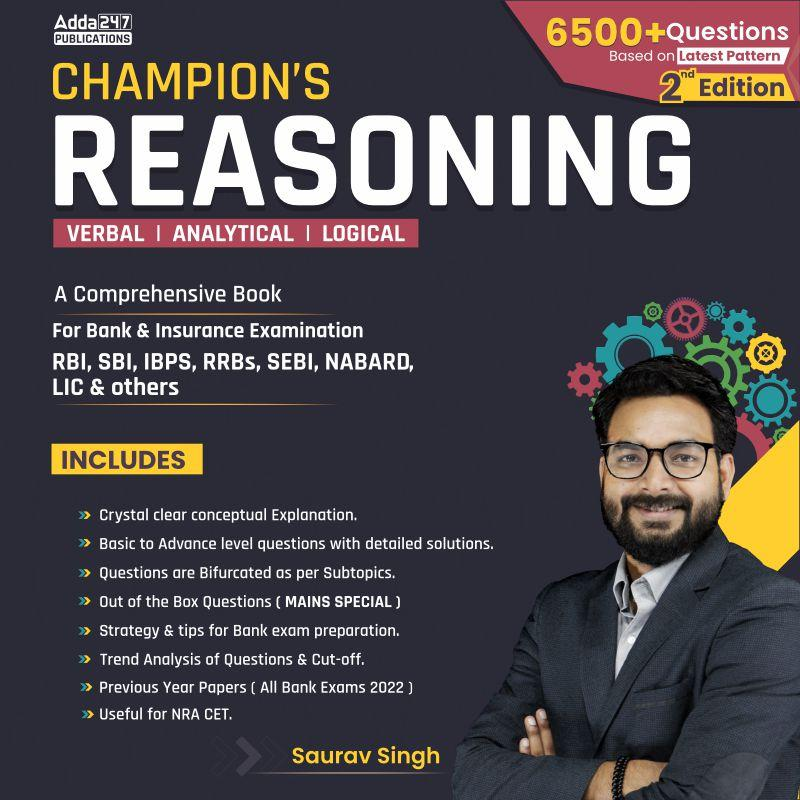Latest Banking jobs   »   IBPS reasoning

# Reasoning Quiz For IBPS PO Prelims 2023-03rd September

Directions (1-5): In the following questions assuming the given statement to be true, find which of the conclusion(s) is/are definitely true and then give your answers accordingly.

Q1. Statements: J < C; A < W ≤ Q; D < O < W; D > C
Conclusions: I. J < W
II. D < Q
(a) Only I is true
(b) Only II is true
(c) Either I or II is true
(d) Neither I nor II is true
(e) Both I and II are true

Q2. Statements: R ≤ D = E; A < Q = R; D < U < V; W > V
Conclusions: I. A < U
II. W > R
(a) Only I is true
(b) Only II is true
(c) Either I or II is true
(d) Neither I nor II is true
(e) Both I and II are true

Q3. Statements: U < O = K; Z = D ≤ A ≤ F; D ≤ O
Conclusions: I. F ≥ K
II. Z ≤ O
(a) Only I is true
(b) Only II is true
(c) Either I or II is true
(d) Neither I nor II is true
(e) Both I and II are true

Q4. Statements: K > Q ≤ U = H, T < P ≥ K < J
Conclusions: I. K > H
II. P > Q
(a) Only I is true
(b) Only II is true
(c) Either I or II is true
(d) Neither I nor II is true
(e) Both I and II are true

Q5. Statements: G = H ≤ I < R, H < T = U
Conclusions: I. U > G
II. R < T
(a) Only I is true
(b) Only II is true
(c) Either I or II is true
(d) Neither I nor II is true
(e) Both I and II are true

Directions (6-10): Study the following information carefully and answer the questions accordingly.

Q R D S T W C K G U V E J Z H I X Y A N O A M P B L G J C E D H G

Q6. How many vowels are immediately preceded and followed by the consonants?
(a) Two
(b) Four
(c) Three
(d) One
(e) Five

Q7. According to the dictionary, what will be the difference in place values of the letters which is ninth from the left end and which is sixteenth from the right end?
(a) 13
(b) 17
(c) 22
(d) 18
(e) None of these

Q8. Which of the following letter is eleventh to the right of tenth letter from the left end?
(a) A
(b) M
(c) O
(d) N
(e) None of these

Q9. How many letters are in between the letter which is fifth from the left end and tenth from the right end according to English dictionary?
(a) 4
(b) 5
(c) 2
(d) 3
(e) None

Q10. If 6th, 10th and 12th letters from the right end and from the left end are changed to their next letter according to English dictionary, then which of the following letter will be twenty fourth from the right end?
(a) K
(b) X
(c) V
(d) F
(e) None of these

Solutions

Solutions (1-5):
S1. Ans. (e)
Sol. I. J < W – True
II. D < Q – True

S2. Ans. (e)
Sol. I. A < U – True
II. W> R – True

S3. Ans. (b)
Sol. I. F ≥ K – False
II. Z ≤ O – True

S4. Ans. (b)
Sol. I. K > H – False
II. P > Q – True

S5. Ans. (a)
Sol. I. U > G – True
II. R < T – False

Solution (6-10):
S6. Ans. (e)
Sol. GUV, VEJ, HIX, YAN, CED

S7. Ans. (d)
Sol. 9th from left end = G, 16th from right end = Y
Required difference of place values= 25 – 7 = 18

S8. Ans. (c)
Sol. Tenth from the left end – U; 11th to the right of U = O

S9. Ans. (d)
Sol. 5th from left end = T, 10th from right end = P
Thus, there are 3 letters (Q, R, S) between P and T in English alphabetic order.

S10. Ans. (c)
Sol. 24th letter from right end is U which is also 10th letter from left end, and U will become V.
Therefore, option (c) is the correct answer.## FAQs

### When will the IBPS PO prelims 2023 be conducted?

IBPS PO Prelims will be conducted on 23, 30 September, and 1 October 2023.

#### Congratulations!Union Budget 2023-24: Free PDF